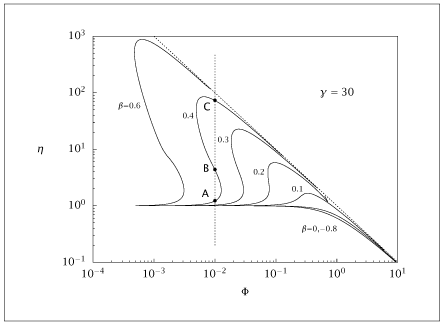## Figure 7.19:

### Effectiveness factor versus normalized Thiele modulus for a first-order reaction in nonisothermal spherical pellet.## Code for Figure 7.19

### main.m

```
1
2
3
4
5
6
7
8
9
10
11
12
13
14
15
16
17
18
19
20
21
22
23
24
25
26
27
28
29
30
31
32
33
34
35
36
37
38
39
40
41
42
43
44
45
46
47
48
49
50
51
52
53
54
55
56
57
58
59
60
61
62
63
64
65
66
67
68
69
70
71
72
73
74
75
76
77
78
79
80
81
82
83
84
85
86
87
88
89
90
91
92
93
94
95
96
97
98
99
100
101
102
103
104
105
106
107
108
109
110
111
112
113
114
115
116
117
118
119
120
121
122
123
124
125
126
127
128
129
130
131
132%Parameterized seaching method, including iterating beta
%
% Picks an initial phi, runs collocation to get a profile and
% uses that to calc eta.
% Then finds a point (phi,eta) along the curve that is some
% predetermined DISTANCE from the previous point
% The variable psi is the angle of the step along a curve with respect
% to the x-axis
% The radius of search rE is on the log scale, it is the radius of search
% on the actual figure, which is then convered to linear scale.

% Written by Brad Schwab, UW, 5/2018.
% Slight editing by jbr, 5/2018.
%

% Struct p contains the following pararmeters:
% R A B phi_prev phi_ini eta_prev gamma beta cS rE I

%tic

%Changeable parameters for optimization
p.gamma = 30;
n = 60; %number of collocation points
npoint = 75; %max number of steps along the curve
betavals = [0.6; 0.4; 0.3; 0.2; 0.1; 0;  -0.8];
rE0 = .15*ones(length(betavals),1);
rE0(5) = .1; %Search will skip the top of a curve at rE0=.15  for beta=0

%Shape factor and boundary condition
p.cS = 1;

%Adds aysmptotic line and verticle line seen in book Figure 7.19
xline1 = [.001 10];
yline1 = [1000 .1];
xline2 = [.01 .01];
yline2 = [.2 500];

%Suppress output from fsolve
options = optimset('Display','off');

%Get collocation points, note the same n is used for all beta/phi values
[R, A, B, Q] = colloc (n-2, 'left', 'right');

%Storage of (phi,eta) values and initializes first run
nbeta = numel(betavals);
phistore =  cell(nbeta,1);
etastore =  cell(nbeta,1);
p.phi_ini = 5E-4;
para_end = .01;
%Initial guesses for fsolve
In01 = ones(n,1); %need an inital eta to start parametric search

for j = 1:nbeta
p.beta = betavals(j);
p.I = calcI(p.gamma, p.beta);
p.rE = rE0(j); %initial radius is set to base, changed in loop
%Phi is fixed at phi_ini for initial search
p.phi_prev = p.phi_ini; %start searching at phi = .01, changed in loop
psi_prev = 0;
delpsi = 0;
In0 = [ones(n,1); 0];

for i = 1:npoint

if (i == 1)
%First need to find an eta for the initial phi
[ini, resid, info] = fsolve(@(x) eta_initial(x,p), In01, options);
deriv = p.A*ini;
last_der = deriv(end);
p.eta_ini = last_der/(p.phi_ini*p.I)^2;
%Set storage variables for first search
p.eta_prev = p.eta_ini;
phistore{j}(i) = p.phi_ini;
etastore{j}(i) = p.eta_ini;
end%if

%Next the parametric search starts
[x, resid, info] = fsolve(@(x) eta_calc(x,p), In0, options);
psi = x(end);
eta = 10^(p.rE*sin(psi) + log10(p.eta_prev));
phi = 10^(p.rE*cos(psi) + log10(p.phi_prev));
In0 = x; %Next set of c are the final values of last set
In0(end) = In0(end) + delpsi; %Next psi is calculated as a fucntion
%of the last two psi values
%Set storage values for this i
phistore{j}(i) = phi;
etastore{j}(i) = eta;
p.phi_prev = phi;
p.eta_prev = eta;
delpsi = psi - psi_prev;
psi_prev = psi;

%Dynamically change radius of search based on change in psi of
%last seach rE in (0,rE0)
p.rE = rE0(j)*(1-abs((delpsi)/pi))^20;

if (abs(log10(p.eta_prev*p.phi_prev)) < para_end)
break
end%if
end%for

table{j} = [phistore{j}; etastore{j}]';
end%for

table2 = [xline1(:), yline1(:), xline2(:), yline2(:)];

save wh.dat table table2

if (~ strcmp (getenv ('OMIT_PLOTS'), 'true')) % PLOTTING
%Labels on main figure
xlabel('phi')
ylabel('eta')
axis([1e-4, 10, 0.1, 1e3])
hold on
for i = 1:nbeta
loglog (table{i}(:,1), table{i}(:,2));
end
loglog (table2(:,1), table2(:,2))
loglog (table2(:,3), table2(:,4))
end % PLOTTING

```

### eta_calc.m

```
1
2
3
4
5
6
7
8
9
10
11
12
13
14
15
16
17
18function retval = eta_calc(para, p)
c = para(1:end-1);
psi = para(end);
eta = 10^(p.rE*sin(psi) + log10(p.eta_prev));
phi = 10^(p.rE*cos(psi) + log10(p.phi_prev));
phi_hat = phi*p.I;

ep = exp(p.gamma*p.beta*(1-c)./(1+p.beta*(1-c)));

retval = zeros(length(para),1);

retval(1:end-1) = p.A*c*2./p.R + p.B*c - phi_hat^2*c.*ep;
retval(1) = p.A(1,:)*c;
retval(end-1) = c(end) - p.cS;

dcdr = p.A*c;
eta_colloc = dcdr(end)/phi_hat^2;
retval(end) = eta - eta_colloc;

```

### eta_initial.m

```
1
2
3
4
5
6
7
8function retval = eta_initial(c, p)
phi_hat = p.phi_ini*p.I;

ep = exp(p.gamma*p.beta*(1-c)./(1+p.beta*(1-c)));

retval = p.A*c*2./p.R + p.B*c - phi_hat^2*c.*ep;
retval(1) = p.A(1,:)*c;
retval(end) = c(end) - p.cS;

```

### indef.m

```
1
2
3
4
5function y = indef(x, a, b)
expi = -(expint(-a/(b*(x-1)-1)) + pi()*i());
y = (exp(a)*(b*x*exp(a/(b*(x-1)-1))*(a+b*x) - a*(a+2*b+2) ...
*expi) - (b+1)*(a+b+1)*exp(a*(1/(b*(x-1)-1)+1))) ...
/2/b/b;

```

### calcI.m

```
1
2
3
4
5
6function aye = calcI(gam, beta)
if beta == 0
aye = 1;
else
aye = sqrt(2*(indef(1, gam, beta) - indef(0, gam, beta)));
end%if

```